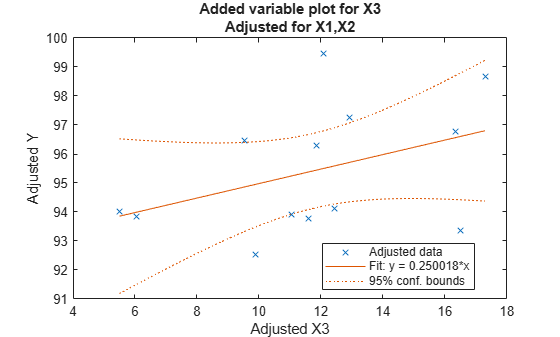Main Content

# addedvarplot

Create added variable plot using input data

## Syntax

```addedvarplot(X,y,num,inmodel) addedvarplot(X,y,num,inmodel,stats) addedvarplot(ax,___) ```

## Description

`addedvarplot(X,y,num,inmodel)` displays an added variable plot using the predictive terms in `X`, the response values in `y`, the added term in column `num` of `X`, and the model with current terms specified by `inmodel`. `X` is an n-by-p matrix of n observations of p predictive terms. `y` is vector of n response values. `num` is a scalar index specifying the column of `X` with the term to be added. `inmodel` is a logical vector of p elements specifying the columns of `X` in the current model. By default, all elements of `inmodel` are `false`.

Note

`addedvarplot` automatically includes a constant term in all models. Do not enter a column of 1s directly into `X`.

`addedvarplot(X,y,num,inmodel,stats)` uses the `stats` output from the `stepwisefit` function to improve the efficiency of repeated calls to `addedvarplot`. Otherwise, this syntax is equivalent to the previous syntax.

`addedvarplot(ax,___)` creates the plot in the axes specified by `ax` instead of the current axes (`gca`). The option `ax` can precede any of the input argument combinations in the previous syntaxes. For more information on creating an `Axes` object, see `axes` and `gca`.

Added variable plots are used to determine the unique effect of adding a new term to a multilinear model. The plot shows the relationship between the part of the response unexplained by terms already in the model and the part of the new term unexplained by terms already in the model. The “unexplained” parts are measured by the residuals of the respective regressions. A scatter of the residuals from the two regressions forms the added variable plot. In addition to the scatter of residuals, the plot produced by `addedvarplot` shows 95% confidence intervals on predictions from the fitted line. The slope of the fitted line is the coefficient that the new term would have if it were added to the model with terms `inmodel`. For more details, see Added Variable Plot.

Added variable plots are sometimes known as partial regression leverage plots.

## Examples

collapse all

Load the data in `hald.mat`, which contains observations of the reaction to heat for various cement mixtures.

```load hald whos```
``` Name Size Bytes Class Attributes Description 22x58 2552 char hald 13x5 520 double heat 13x1 104 double ingredients 13x4 416 double ```

Create an added variable plot to investigate the effect of adding the third column of `ingredients` to a model that contains the first two columns.

```inmodel = [true true false false]; addedvarplot(ingredients,heat,3,inmodel)```The wide scatter plot and the low slope of the fitted line are evidence against the statistical significance of adding the third column to the model.

## Alternative Functionality

You can create a linear regression model object `LinearModel` by using `fitlm` or `stepwiselm` and use the object function `plotAdded` to create an added variable plot.

A `LinearModel` object provides the object properties and the object functions to investigate a fitted linear regression model. The object properties include information about coefficient estimates, summary statistics, fitting method, and input data. Use the object functions to predict responses and to modify, evaluate, and visualize the linear regression model.

## See Also

Introduced before R2006a

Download ebook